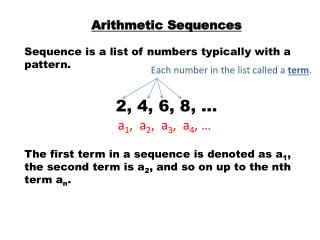Download PresentationArithmetic Sequences Sequence is a list of numbers typically with a pattern. 2, 4, 6, 8, …

Arithmetic Sequences Sequence is a list of numbers typically with a pattern. 2, 4, 6, 8, … - PowerPoint PPT Presentation

Arithmetic Sequences Sequence is a list of numbers typically with a pattern. 2, 4, 6, 8, … The first term in a sequence is denoted as a 1 , the second term is a 2 , and so on up to the nth term a n. Each number in the list called a term. a 1 , a 2 , a 3 , a 4 , ….I am the owner, or an agent authorized to act on behalf of the owner, of the copyrighted work described.
Download PresentationArithmetic Sequences Sequence is a list of numbers typically with a pattern. 2, 4, 6, 8, …

Download Policy: Content on the Website is provided to you AS IS for your information and personal use and may not be sold / licensed / shared on other websites without getting consent from its author.While downloading, if for some reason you are not able to download a presentation, the publisher may have deleted the file from their server.

- - - - - - - - - - - - - - - - - - - - - - - - - - E N D - - - - - - - - - - - - - - - - - - - - - - - - - -
Presentation Transcript
1. Arithmetic Sequences • Sequence is a list of numbers typically with a pattern. • 2, 4, 6, 8, … • The first term in a sequence is denoted as a1, the second term is a2, and so on up to the nth term an. Each number in the list called a term. a1, a2, a3, a4, …

2. Finite Sequence has a fixed number of terms. {2, 4, 6, 8} A sequence that has infinitely many terms is called an infinite sequence. {2, 4, 6, 8,…} Algebraically, a sequence can be written as an explicit formula or as a recursive formula. Explicit formulas show how to find a specific term number (n). Recursive formula show how to get from a given term (an-1) to the next term (an)

3. An Arithmetic Sequence is a sequence where you use repeated addition (with same number) to get from one term to the next. Ex: 4, 1, -2, -5, … is an arithmetic sequence -3 -3 -3 The number that needs to be added each time to get to the next term is called the common difference The common difference for the above arithmetic sequence is -3 .

4. Explicit formula for Arithmetic Sequence: an= + (n - 1)d Recursive formula for an Arithmetic Sequence: a1 = # an = an-1 + d First term Common difference For the example: 4, 1, -2, -5, … Explicit Formula Substitute the values: an= 4 + (n – 1)(- 3) So the explicit formula is: an= -3n + 7 The Recursive Formula is: a1= 4 an = an-1 – 3

5. How do you add these sequences of numbers? A series is the sum of ALL the terms of a sequence. (can be finite or infinite) A partial sum is the sum of the first n terms of a series…denoted Sn First term Last term Number of terms

6. For the example: 4, 1, -2, -5, … 1) Find S4. Find S20. (Think….)

7. Example: For the arithmetic sequence 2, 6, 10, 14, 18, … Write the explicit formula for the sequence. Write the recursive formula for the sequence. c) Find the 15th partial sum of the sequence (S15). a1 = # an = an-1 + d an =